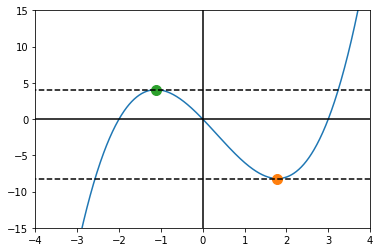Learning library

For all the self-taught geeks out there, here is our content library with most of the learning materials we have produced throughout the years.

It makes sense to start learning by reading and watching videos about fundamentals and how things work.

Data Science and Machine Learning - 16 wks

Full-Stack Software Developer - 16w

Search from all Lessons

Social & live learning

The most efficient way to learn: Join a cohort with classmates just like you, live streams, impromptu coding sessions, live tutorials with real experts, and stay motivated.

← Back to Lessons
Edit on Github
Open in Collab

# Introduction to Derivatives¶

In :
import matplotlib.pyplot as plt
import numpy as np
import sympy as sy


## Motivation: Slope of a Line¶

$slope = \frac{Y2 - Y1}{X2 - X1}$

Consider:

$f(x) = x + 1 \ \ and \ \ g(x) = x^2 + 1$
In :
def f(x): return x + 1
def g(x): return x**2 + 1
x = np.linspace(-2,2,100)

In :
plt.plot(x,f(x),label='f')
plt.plot(x,g(x),label='g')
plt.plot(0,f(0),'ro')
plt.plot(1,f(1),'ro')
plt.legend()

Out:
<matplotlib.legend.Legend at 0x7f17c94f31f0>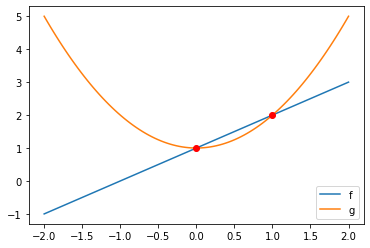In :
x2 = np.linspace(1,1.1)

In :
plt.plot(x2,f(x2),label='f')
plt.plot(x2,g(x2),label='g')
plt.legend()
plt.title('Zooming in on $x = [1,1.1]$\nNow they both look Linear!')

Out:
Text(0.5, 1.0, 'Zooming in on $x = [1,1.1]$\nNow they both look Linear!')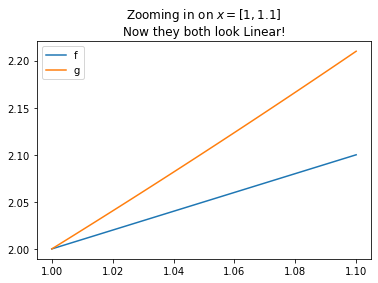In :
x2 = np.linspace(1,1.001,100)
plt.plot(x2,f(x2),label='f')
plt.plot(x2,g(x2),label='g')
plt.legend()
plt.title('Zooming in on $x = [1,1.001,100]$\nNow they both look Linear!')

Out:
Text(0.5, 1.0, 'Zooming in on $x = [1,1.001,100]$\nNow they both look Linear!')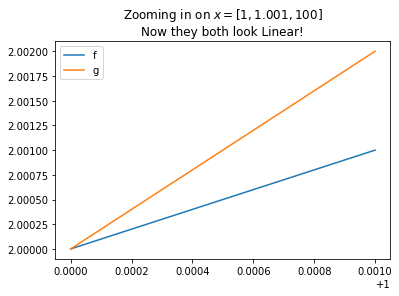Definition

$f'(x) = \lim_{h \to 0} \frac{f(x + h) - f(x)}{h} \$

Note: h is the distance, gets smaller and smaller so we can get the smalles possible variation of x (slope)

We can interpret the derivative in a few ways. As we started looking at the difference between terms, we can consider the derivative as a measure of the rate of change of some sequence of numbers.

Similarly, if we have a closed form sequence rather than a sequence we can regard the derivative as a function that represents the rate of change of our original function.

## Derivative as Function with Python¶

First, we can investigate the derivative of a function using Sympy's diff function. If we enter a symbolic expression $f$ in terms of some variable $x$, we will be able to get the derivative of this expression with sy.diff(f, x). A simple example follows.

In :
def f(x):
return x**2 - 2*x + 1

In :
def df(x):
h = 0.000001
return (f(x + h) - f(x))/h

In :
x = np.linspace(-4, 4, 1000)
plt.plot(x, f(x), label = '$f(x)$')
plt.plot(x, df(x), label = '$f\'(x)$')
plt.axhline(color = 'black')
plt.axvline(color = 'black')
plt.legend(loc = 'best', frameon = False)
plt.title("Function and its Derivative")

Out:
Text(0.5, 1.0, 'Function and its Derivative')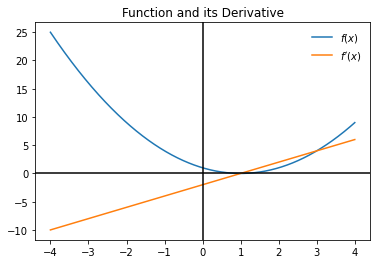## A function a its derivative¶

In :
x = np.linspace(-5, 2, 1000)
plt.plot(x, f(x), label = '$f(x)$')
plt.plot(x, df(x), label = '$f\'(x)$')
plt.axhline(color = 'black')
plt.axvline(color = 'black')
plt.legend(frameon = False)
plt.title("Another function and its Derivative")

Out:
Text(0.5, 1.0, 'Another function and its Derivative')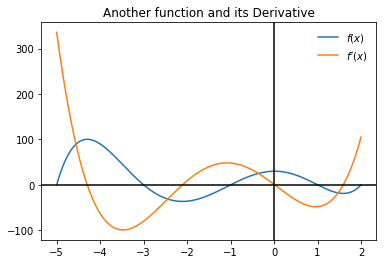We can glean important information from the plot of the derivative about the behavior of the function we are investigating, particularly the maximum and minimum values. Perhaps we would only have the plot of the derivative, can you tell where the max and min values occur?

In :
x = np.linspace(-5, 2, 1000)
plt.plot(x, df(x), label = '$f\'(x)$')
plt.axhline(color = 'black')
plt.axvline(color = 'black')
plt.legend(frameon = False)
plt.title("Just the Derivative")

Out:
Text(0.5, 1.0, 'Just the Derivative')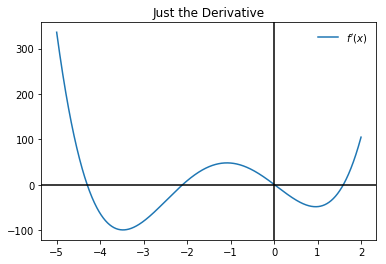In :
x = sy.Symbol('x')
df = sy.diff(f(x), x)
df = sy.expand(df)
df

Out:
5*x**4 + 24*x**3 - 6*x**2 - 72*x + 1

# Derivative as Tangent Line¶

We can also interpret the derivative as the slope of a tangent line, or better yet an approximation for this tangent line. For example, the derivative at some point $a$ can be thought of as the slope of the line through $a$ and some other point $a + \Delta x$ where $\Delta x$ is very small.

Again, we would have something like:

$f'(a) = \frac{f(a + \Delta x) - f(a)}{\Delta x}$

for some arbitrarily small value of $\Delta x$. We can also understand the expression above as providing us a means of approximating values close to $x = a$ using the tangent line. If we rearrange terms, notice:

$f(a + \Delta x) = f'(a)\Delta x + f(a)$

What this does, is tells us the slope of the line tangent to the graph at the point $(a, f(a))$. Suppose we have the function $f(x) = x^2$, and we want to know the slope of the tangent line at $x = 2$. We can define a function as usual, and a function for the derivative. We can use these to write an equation of the line tangent to the graph of the function at this point.

In :
def f(x):
return x**2

def df(x):
h = 0.00001
return (f(x + h) - f(x))/h

In :
df(2)

Out:
4.000010000027032
In :
def tan_plot(a):
x = np.linspace((a-4), (a+4), 1000)
y = df(a)*(x - a) + f(a)
plt.plot(x, f(x))
plt.plot(a, f(a), 'o', markersize = 10)
plt.plot(x, y, '--k')
plt.axhline(color = 'black')
plt.axvline(color = 'black')

In :
tan_plot(2)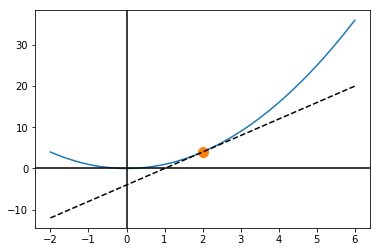In :
def g(x):
return x*(x+2)*(x - 3)

def dg(x):
h = 0.000001
return ((g(x+h)-g(x))/h)

In :
x = np.linspace(-3, 4, 1000)
plt.plot(x, g(x))
plt.axhline(color = 'black')
plt.axvline(color = 'black')

Out:
<matplotlib.lines.Line2D at 0x11dc14e80>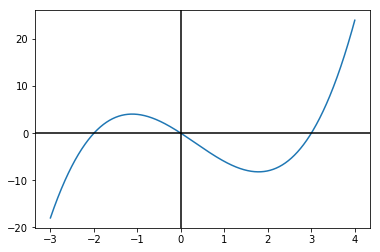In :
x = sy.Symbol('x')
df = sy.diff(g(x), x)
df = sy.simplify(df)
a, b = sy.solve(df, x)

In :
a, b

Out:
(1/3 + sqrt(19)/3, -sqrt(19)/3 + 1/3)
In :
x = np.linspace(-3, 4, 1000)
plt.plot(x, g(x))
plt.axhline(color = 'black')
plt.axvline(color = 'black')
plt.plot(a, g(a), 'o')
plt.plot(b, g(b), 'o')

Out:
[<matplotlib.lines.Line2D at 0x11e006898>]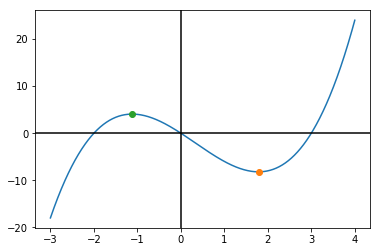In :
def tan_plot(a, b):
x = np.linspace(-5,5, 1000)
y1 = dg(a)*(x - a) + g(a)
y2 = dg(b)*(x - b) + g(b)
plt.plot(x, g(x))
plt.plot(a, g(a), 'o', markersize = 10)
plt.plot(b, g(b), 'o', markersize = 10)
plt.plot(x, y1, '--k')
plt.plot(x, y2, '--k')
plt.ylim(-15, 15)
plt.xlim(-4,4)
plt.axhline(color = 'black')
plt.axvline(color = 'black')

In :
tan_plot(a, b)